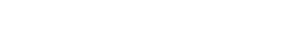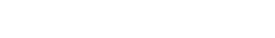## Chemistry Paper 1 - Alliance High School Pre-Trial Examination 2018

ALLIANCE HIGH SCHOOL
CHEMISTRY PRE-TRIAL
PAPER 1
233/1 - 2018
TIME: 2 HOURS

1. A mixture consists of sulphur and iron fillings, the mixture is heated in an open crucible.
1. State the observation. (1 mk)
2. Write an equation for the reaction. (1 mk)
2. Element K (not actual symbol of the element) has isotopes with relative abundance as shown below.

 Isotope Relative Abundance (%) 105K 18.69 115K 81.28

Calculate the relative atomic mass of K. (2 mks)
3. The table below gives the ionization energies of the alkali metals.
 Element 1stionization energyKJmol- A 494 B 418 C 519

Which of the three metals is the least reactive. Give a reason. (1 mk)
4. Dry hydrogen gas was passed over heated oxide of copper weighing 14.3g, at the end of the experiment 12.7g of copper and 1.8g of water were obtained.
1. Determine the formula of the oxide. (Cu - 63.5, O - 16) (2 mks)
2. Name two other gases tha can be used instead of hydrogen. (1 mk)
5. A piece of burning Magnesium was introduced into a gas jar of nitrogen, water was then added to the products. The resultant solution was tested with litmus paper.
1. Explain the observation. (1 mk)
2. Write an equation for the formation of the final solution. (1 mk)
6. State one reagent that can be used to distinguish between the pairs of ions.
1. Pb2+(aq) Al3+(aq) (2 mks)
2. SO2-4 and SO2- (2 mks)
7. 20cm3 of sodium carbonate solution was reacted completely with 25cm3 of a 0.8M hydrochloric acid according to the equations.
Na2CO3 + 2HCl --------> 2NaCl + CO2 + H2O
Calculate the concentrationn of sodium carbonate in grams per litre. (3 mks)
8. The electronic arrangement of ions of a certain element represented by letters P Q R and S
P2- 2:8:8
Q2+ 2:8
R+ 2:8
S 2:8:8
1. Explain why S is not represented as an ion. (1 mk)
2. Which element has the largest atomic radius? Explain. (1 mk)
9. A label on a bottle of Hydrochloric acid has the following information;
Density 1.134gm-3 and percentage purity 37%
Determine the molarity of the solution. (4 mks)
10. The following figure shows a section of apparatus used in laboratory for measuring volume.1. Identify the apparatus and state the correct reading of volume. (2 mks)
11. The atomic number of P and S are 6 and 17 respectively.
1. Using dots and cross, draw the compound formed when P reacts with S. (1 mk)
2. Name the type of bond and explain whether the compound would conduct electricity. (2 mks)
12. A given volume of a gas G diffuses through a membrane in 10 seconds. Under same condition, an equal volume of oxygen diffuses for 12.5 sec. Determine the molecular mass of G. (2 mks)
1. Using an equation, explain the observation made when sodium hydroxide is added to Aluminum oxide dropwise until in excess. (2 mks)
2. Name the product of the reaction. (1 mk)
13. Cynogen is a gaseous compound of carbon and Nitrogen only. On complete combustion in oxygen, 250cm3. Determine the formula of cynogen. (3 mks)
14. A stream of Ammonia was bubbled in water containing litums papers.1. State one physical property of the gas. (1 mk)
2. Explain the observation made during the experiment. (1 mk)
15. A student was given solid potassium permanganate to prepare a colourless gas.
1. Draw a complete set up to carry out the experiment naming the gas. (3 mks)
16. Propene and propane both decolourises bromine liquid at different conditions.
1. Explain with an equation how the hydrocarbons decolourises bromine. (4 mks)
2. Complete the reaction by indicating the polymer. (1 mk)
C2H4 ------>
3. State type of reaction and calculate the value of n given the molecular mass of polymer is 33600. (4 mks)
17. In the apparatus shown in the diagram, compound M and N are reacted to produce Ammonia which is passed to T where it is burnt.1. Name the substance M and N. (2 mks)
2. Role of cotton wool and calcium oxide. (1 mk)
3. Write an equation for the reaction. (2 mks)
18. Below is a chemical reaction.1. Using an energy level diagram, represent the reaction when vanandium(V)oxide is used. (2 mks)
2. State the effect of increase in temperature to the equilibrium. (1 mk)
3. Give one characterisitc of a dynamic equilibrium. (1 mk)
19. Study the test below and answer the questions.
 Test Observation (i) P is heated until no further change A colourless liquidcondensed on the coolerparts of the test tube-A colourless gas which turnsAqueous potassium chromate(VI) green was given out and redbrown residue R was left. (ii)Chlorine gas was bubbledthrough an acqueous of P Solution turn from green to yellow

1. Identify P and R (1 mk)
2. Write an equation for test (i) (1 mk)
3. Describe how a student would test for anion in solid P. (3 mks)
4. Name one reagent that can be used to confirm cation in P. (1 mk)
20. Name the main ores of: Iron, copper, sodium, aluminium (2 mks)
21. Calculate the oxidation number of P given the following P2O5 (1 mk)
22. Identify z and y given the following equation. (3 mks)23. State and explain observation made when chlorine gas is bubbled through a solution of potassium iodide. (2 mks)
24. Sketch the bond formed between the complex tetramine copper (II) ions.
(1 mk)
25. Explain why graphite is used as a lubricant in machines. (3 mks)
26. Describe how you would prepare crystals of sodium nitrate starting with 200cm3 of 2M sodium hydroxide. (3 mks)
27. One liter of a gas has a mass of 1.16g at s.t.p. Analysis shows that this gas contains 92.3% carbon and 7.69 hydrogen. Calculate the molecular formular of the gas. (C = 12.0, H = 1.0) (2 mks)
28. Below is a diagram of a dry cell. Study it and answer the questions that follows.1. Write an equation at anode and cathode. (2 mks)
2. Why is Ammonium Chloride used as a paste rather than a dry solid. (1/2 mark)
29.
1. Identify the cleansing agent. (1/2 mark)
2. Write the molelular formular of the cleansing agent above. (1/2 mark)
3. State one advantage of using the cleansing agent. (1/2 mark)
30. A student set up the electrochemical  cell as represented below.
Pb(s)/Pb(NO3)(l) 1M // SnCl2(aq) (1M)/Sn(s)
1. State the condition under which the set up was made. (1 mk)
2. Using the Eθ given below, calculate the e.m.f of the cell. (1 mk)
Pb(aq)2+ + 2e- -----> Pb(s) -- 0.13v
Sn(aq)2+ + 2e- -----> Sn(s) -- 0.14v

#### Download Chemistry Paper 1 - Alliance High School Pre-Trial Examination 2018.

• ✔ To read offline at any time.
• ✔ To Print at your convenience
• ✔ Share Easily with Friends / Students